# 3. Consider a perfectly inelastic supply curve at q = 1,013, and a perfectly elastic demand...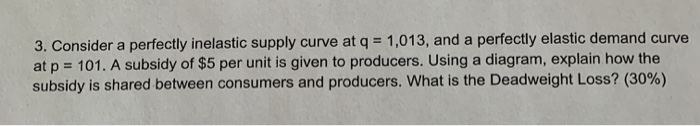3. Consider a perfectly inelastic supply curve at q = 1,013, and a perfectly elastic demand curve at p = 101. A subsidy of \$5 per unit is given to producers. Using a diagram, explain how the subsidy is shared between consumers and producers. What is the Deadweight Loss? (30%)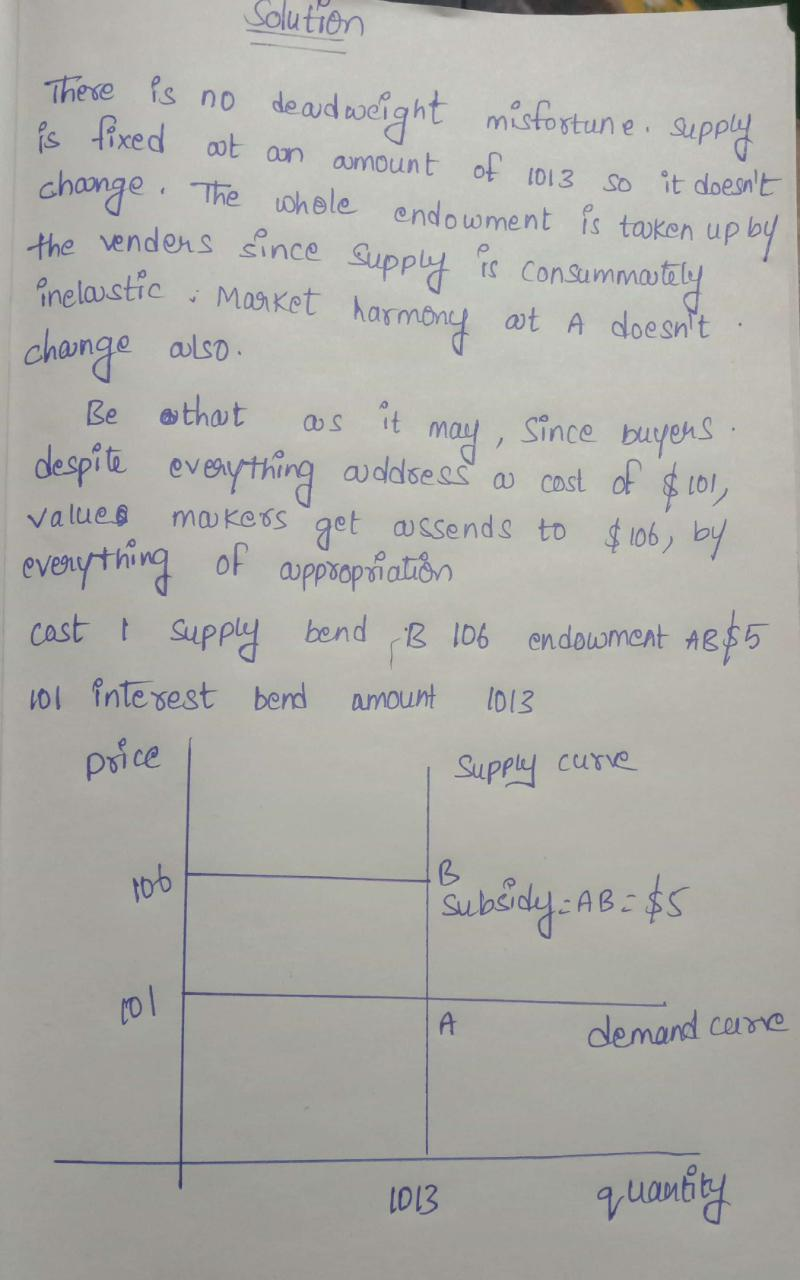##### Add Answer of: 3. Consider a perfectly inelastic supply curve at q = 1,013, and a perfectly elastic demand...
Similar Homework Help Questions
• ### Question 1: In a perfectly competitive market, the demand curve is given as: Q=100-5P, the supply curve is given as Q=3P...

Question 1: In a perfectly competitive market, the demand curve is given as: Q=100-5P, the supply curve is given as Q=3P-12. Compute the total social surplus of this market. If the government impose a tax on the producers, and the tax rate is \$2 per unit produced. What is the deadweight loss? If the government impose a tax on the consumers, and the tax rate is \$2 per unit purchased, graphically show the change in the market equilibrium and the...

• ### Question 1 (45%): In a perfectly competitive market, the demand curve is given as: Q=100-5P, the supply curve is given...

Question 1 (45%): In a perfectly competitive market, the demand curve is given as: Q=100-5P, the supply curve is given as Q=3P-12. I. Compute the total social surplus of this market. (10%) II. If the government impose a tax on the producers, and the tax rate is \$2 per unit produced. What is the deadweight loss? (10%) III. If the government impose a tax on the consumers, and the tax rate is \$2 per unit purchased, graphically show the change...

• ### 4. Consider a perfectly elastic supply curve, at p = 10, along with a perfectly inelastic...

4. Consider a perfectly elastic supply curve, at p = 10, along with a perfectly inelastic demand curve, at q = 103. Calculate the Consumer Surplus and the Producer Surplus in this market. Show your work. (10%)

• ### Q3) Suppose that the market demand and supply curve in a competitive market are Q"-15 - 2P and QS...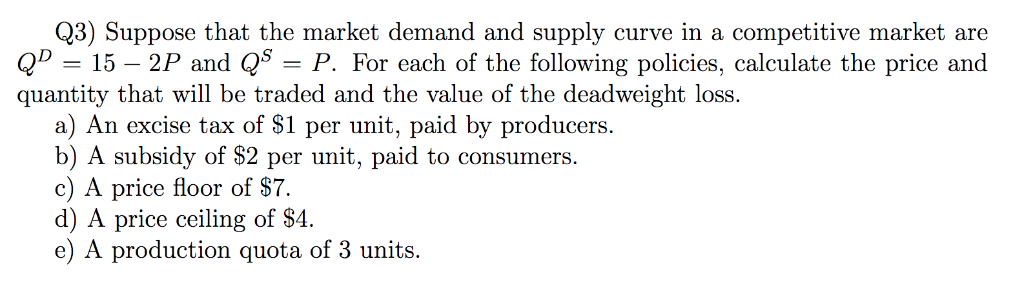Q3) Suppose that the market demand and supply curve in a competitive market are Q"-15 - 2P and QS-P. For each of the following policies, calculate the price and quantity that will be traded and the value of the deadweight loss. a) An excise tax of S1 per unit, paid by producers. b) A subsidy of \$2 per unit, paid to consumers. c) A price floor of S7. d) A price ceiling of S4. e) A production quota of 3...

• ### Consider a perfectly competitive market where the market demand curve is given by Q = 76−8P...

Consider a perfectly competitive market where the market demand curve is given by Q = 76−8P and the market supply curve is given by Q=−8+4P. In situations （c）, determine the following items (i-viii) (c) A market with subsidy S=9. i) The quantity sold in the market. ii) The price that consumers pay (before all taxes/subsidies). iii) The price that producers receive (after all taxes/subsidies). iv) The range of possible consumer surplus values. v) The range of possible producer surplus values....

• ### 3. (20%) Consider the market was unregulated, with an equilibrium price of \$200, and a quantity of 20,000 The suppl...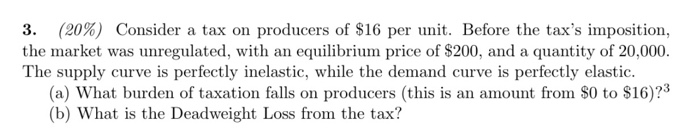3. (20%) Consider the market was unregulated, with an equilibrium price of \$200, and a quantity of 20,000 The supply curve is perfectly inelastic, while the demand curve is perfectly elastic. (a) What burden of taxation falls on producers (this is an amount from \$0 to \$16)?3 (b) What is the Deadweight Loss from the tax? a tax on producers of \$16 per unit. Before the tax's imposition, 3. (20%) Consider the market was unregulated, with an equilibrium price of...

• ### 10.19. In a perfectly competitive market, the market demand curve is Qd = 10 -p, and...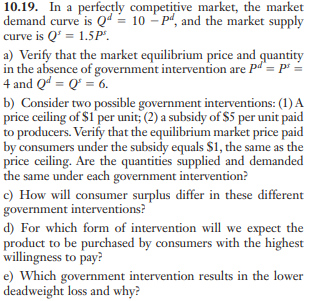10.19. In a perfectly competitive market, the market demand curve is Qd = 10 -p, and the market supply curve is Q 1.5P a) Verify that the market equilibrium price and quantity in the absence of government intervention are Pd= P 4 and Qd Q 6. b) Consider two possible government interventions: (1)A price ceiling of \$I per unit; (2) a subsidy of \$5 per unit paid to producers. Verify that the equilibrium market price paid by consumers under the...

• ### A monopoly has A. A perfectly elastic demand curve B. A perfectly elastic supply curve C....

A monopoly has A. A perfectly elastic demand curve B. A perfectly elastic supply curve C. An inelastic demand curve D. less elastic demand curve than a competitive firm

• ### 1. Consider a perfectly competitive market where the market demand curve is given by Q 72-4P and ...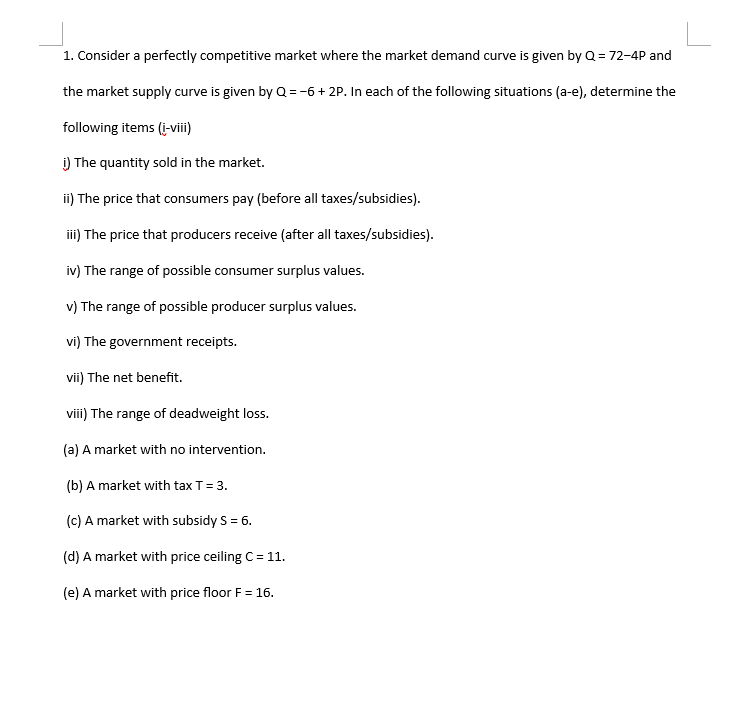Please answer question B 1. Consider a perfectly competitive market where the market demand curve is given by Q 72-4P and the market supply curve is given by Q-6+2P. In each of the following situations (a-e), determine the following items (i-vili) ) The quantity sold in the market. ii) The price that consumers pay (before all taxes/subsidies) ili) The price that producers receive (after all taxes/subsidies). iv) The range of possible consumer surplus values. v) The range of possible producer...

• ### 33. If the short-run supply curve for fresh fruit is perfectly inelastic and the demand curve...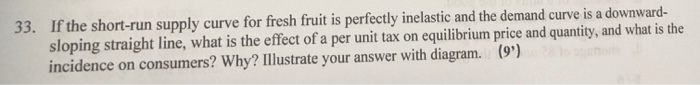33. If the short-run supply curve for fresh fruit is perfectly inelastic and the demand curve is a downward- sloping straight line, what is the effect of a per unit tax on equilibrium price and quantity, and what is the incidence on consumers? Why? Illustrate your answer with diagram.(9')

Need Online Homework Help?